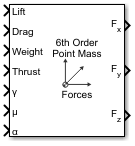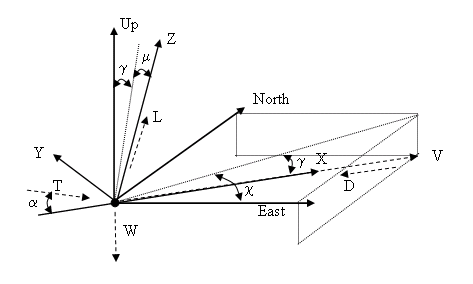# 6th Order Point Mass Forces (Coordinated Flight)

Calculate forces used by sixth-order point mass in coordinated flight

•Libraries:
Aerospace Blockset / Equations of Motion / Point Mass

## Description

The 6th Order Point Mass Forces (Coordinated Flight) block calculates the applied forces for a single point mass or multiple point masses. For more information on the system for the applied forces, see Algorithms.

## Limitations

• The block assumes that there is fully coordinated flight, i.e., there is no side force (wind axes) and sideslip is always zero.

• The flat Earth reference frame is considered inertial, an approximation that allows the forces due to the Earth motion relative to the "fixed stars" to be neglected.

## Ports

### Input

expand all

Lift, specified as a scalar or array, in units of force.

Data Types: `double`

Drag, specified as a scalar or array, in units of force.

Data Types: `double`

Weight, specified as a scalar or array, in units of force.

Data Types: `double`

Thrust, specified as a scalar or array, in units of force.

Data Types: `double`

Flight path angle, specified as a scalar or array, in radians.

Data Types: `double`

Bank angle, specified as a scalar or array, in radians.

Data Types: `double`

Angle of attack, specified as a scalar or array, in radians.

Data Types: `double`

### Output

expand all

Force in x-axis, specified as a scalar or array, in units of force.

Data Types: `double`

Force in y-axis, specified as a scalar or array, in units of force.

Data Types: `double`

Force in z-axis, specified as a scalar or array, in units of force.

Data Types: `double`

## Algorithms

This figure shows the applied forces in the system used by this block.The applied forces [FxFyFh]T are in a system is defined by x-axis in the direction of vehicle velocity relative to air, z-axis is upwards and y-axis completes the right-handed frame and are functions of lift (L), drag (D), thrust (T), weight (W), flight path angle (γ), angle of attack (α), and bank angle (μ).

`$\begin{array}{l}{F}_{x}=T\mathrm{cos}\alpha -D-W\mathrm{sin}\gamma \\ {F}_{y}=\left(L+T\mathrm{sin}\alpha \right)\mathrm{sin}\mu \\ {F}_{z}=\left(L+T\mathrm{sin}\alpha \right)\mathrm{cos}\mu -W\mathrm{cos}\gamma \end{array}$`

## Version History

Introduced before R2006a